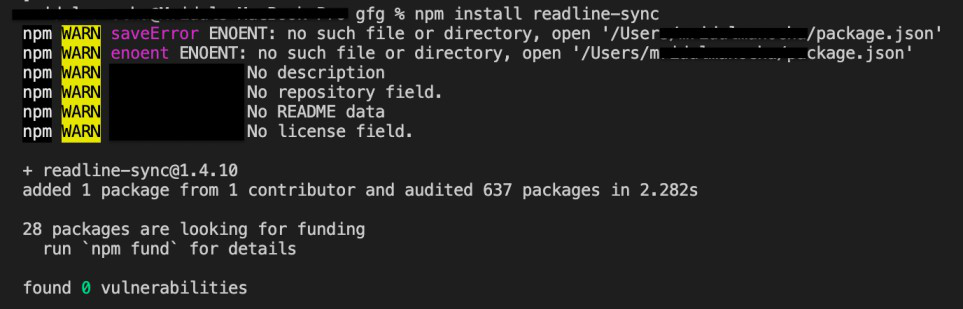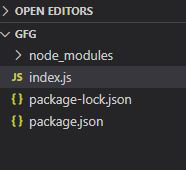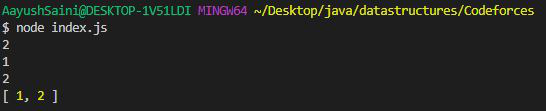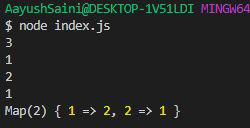Open in App
Not now

# How to take input in Node.js ?

• Difficulty Level : Easy
• Last Updated : 07 Oct, 2021

Nowadays the extension of JavaScript i.e Node.js is quite popular among programmers in the field of competitive programming. In this article, we will discuss how to take input from the user.

readline-sync: This is the third party module that is used for taking the input from the user synchronously. So, the execution of the programs is line by line.

Installing module:

`npm install readline-sync`Project structure:Filename- index.js: Taking input array from the user

## Javascript

 `// Importing the module``const readline = require(``"readline-sync"``);`` ` `// Enter the number``let a = Number(readline.question());``let number = [];``for` `(let i = 0; i < a; ++i) {``  ``number.push(Number(readline.question()));``}``console.log(number);`

Run index.js file using below command:

`node index.js`

Output: This will be in console output.Filename- index.js: Finding the frequency of the numbers

## Javascript

 `// Importing the module``const readline = require(``"readline-sync"``);`` ` `// Enter the number``let a = Number(readline.question());``let number = [];`` ` `// Creating map``let map = ``new` `Map();``for` `(let i = 0; i < a; ++i) {``  ``let number = Number(readline.question());``  ``if` `(map.has(number)) {``    ``map.set(number, map.get(number) + 1);``  ``} ``else` `{``    ``map.set(number, 1);``  ``}``}`` ` `console.log(map);`

Run index.js file using below command:

`node index.js`

Output: This will be in console output.My Personal Notes arrow_drop_up